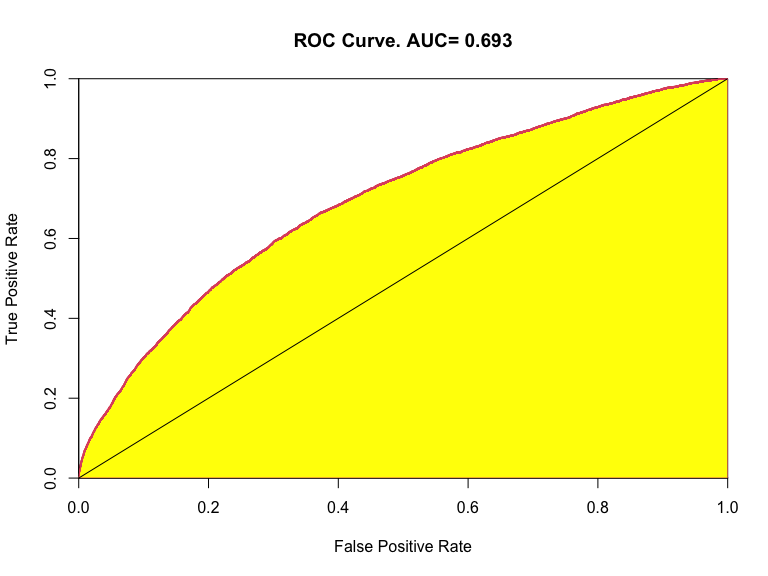# 1 The Receiver Operating Characteristics (ROC) curve

source

  my.auc=function(pred,target){
#### too slow. use auc_roc
# pred is predictor
# target is the true vector (0,1)
# the return value is auc the area under the roc curve
target   =target[order(pred)]
n   =length(target)
totP=sum(target)
totN=n-totP
FN  =cumsum(target)
TN  =(1:n)-FN
FP  =totN-TN
TP  =n-totN-FN
x   =c(1,FP/(TN+FP))
y   =c(1,TP/(FN+TP))
auc =-0.5*sum(diff(x)*(y[-1]+y[-n]))
obj=list(auc=auc,x=x,y=y)
class(obj)      ="auc"

return(obj)
}
plot.auc=function(obj){
with(auc,plot(x,y,type="l",col=2,lwd=3,
xaxs="i",yaxs="i",
xlab="False Positive Rate",
ylab="True Positive Rate",
main=paste("ROC Curve. AUC=",round(obj$auc,3)))) xp=c(auc$x,0,1,1)
yp=c(auc$y,0,0,1) polygon(xp, yp , density = NA, angle = 45, border = 2, col = "yellow") abline(a=0,b=1) } ## 1.1 The problem of a binary classification. Suppose we obtained a continuos scoring from a composition of different explanatory variables, which should help us in deciding if a unit must be classified as 0 or 1. For example we could have obtained it with a linear predictor from a logistic regression. From an estimated linear predictor $$\hat{\eta}_i$$ (whatever the variables used to obtain it), we can compute an estimate of the probability of the $$i-$$th unit of being “1” (or TRUE), according to the inverse logit transform of the estimated predictor: $\hat{p}_i=\hat{Prob}(Y_i=1)=\frac{\exp(\hat{\eta}_i)}{1+\exp(\hat{\eta}_i)}$ which maps a real varable fro $$\cal{R}_1$$ to $$[0,1]$$. Of course low values of $$\hat{\eta}_i$$ (that is negative values with large absolute value) will give estimated probabilities near zero, while large positive values will give values of $$\hat{p}_i$$ near one. This could be enough for our logistic regression model estimation (which possibly has been estimated with glm in R, and possibly chosen after a model selection, through AIC or some sort of Cross Validation), but certainly this is not enough if we mest decide something, that is: • A patient is lickely to be sick? • An on line transaction is honest or is a fraude transaction? • The team “myfavouriteteam” will win a soccer match? ## 1.2 Choosing a threshold. In other terms I want to use the values of $$\hat{p}_i$$ to classify the units $$U_i, i=1,2,...,n$$ in a group (0 for low values) or in another one (1 vor large values) A first simple rule could be: Classify the unit as group “1” (ore TRUE) if $$p_i\ge\frac{1}{2}$$. However this can be measleading in many cases. Suppose this very simple (artificial) situation: source y=c(0,0,0,0,0,0,0,1,1,1) eta=c(-5,-4,-3,-2,-1.5,-1,0.5,1,3,4) p=exp(eta)/(1+exp(eta)) plot(p,y) abline(v=0.5) plot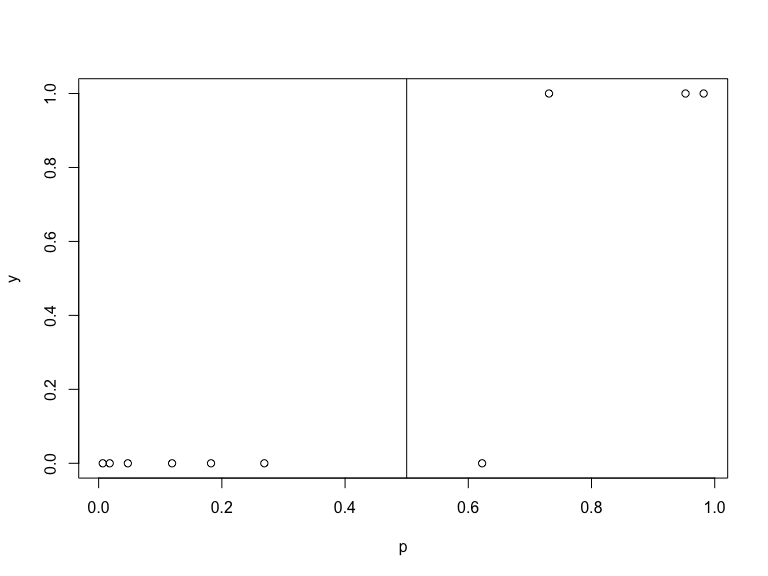source print(round(p,3)) output   0.007 0.018 0.047 0.119 0.182 0.269 0.622 0.731 0.953 0.982 A threshold of 0.5 is too crude, we would classify the sixth unit in the wrong group, while, given the good separation between groups, a threshold $$p_t$$ in the interval $$p_7 < p \le p_8$$, that is 0.622, 0.731, will do the job perfectly, without classification errors. Any other threshold will not be efficient, because will cause at least a unit misclassified (as for $$p_t=0.5$$) Of course reality will not be so confortable (and maybe also boring…): look at another more realistic example (again on artificial data, only to fix ideas). source y=c(0,0,0,1,0,1,0,1,1,1) eta=c(-5,-4,-3.5,-2,-1.5,-1.1,0.5,1,3,4) p=exp(eta)/(1+exp(eta)) plot(p,y) abline(v=0.5) plot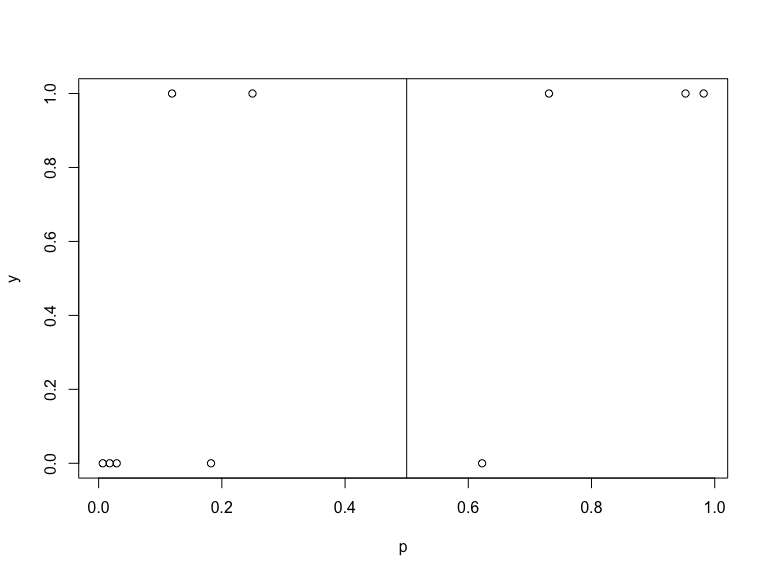Now the situation, even if very simple, is different: there is not a clear separation between groups, and every theshold will give some misclassification error: “0” classified as “1” (false positive, or FP, type I error) or “1” classified as “0” (false negative, or FN, type II error). The first case is when we say: “Stop this transaction: it is a fraude!” and afterwards we discover that it was honest; the second case is the opposite: “Approve this transaction and pay it” and alter we discover that it was a fraud. Or we say that someone is sick while she/he is ok. Of course the kind of errors will have different consequences, or, if we want to quantify with vile money, will have different costs. In the previous example, if we want to be safe from II type errors, but we can bear some type I errors, we could be conservative and fix a threshold greater than $$p_3$$ but less or equal to $$p_4$$, to be sure that all positive unit will be correctly classified, but at the cost of two type II errors. If we moved the threshold on the right we would have errors of both type. On the other extreme, if we choose a threshold greater than $$p_7$$ but less than $$p_8$$ we would have no type II errors but two type I errors… ## 1.3 Some general consideration In a general real life situation, things will be of course more complicated. First of all, if we want to understand the effect of a threshold, it is the some thing to work on the $$\hat{p}$$ scale, or directly on the $$\hat{\eta}$$ scale, or on any other monotone transformation of them… From the last example we see that any threshold less than $$p_3$$ or greter than $$p_8$$ is not efficient. Furthermore, any threshold between consecutive values of $$p_i,p_{i+1}$$ will give same error rates, so that we will have five different configuration of errors. With $$n$$ we cannot have more than $$n+1$$ distinct configurations ## 1.4 A more complicated situation source  l8=glm(isFraud~card4*card6+log(TransactionAmt)+I((log(TransactionAmt))^2) +sin(pi*hour/12) +cos(pi*hour/12) +sin(pi*hour/12)*cos(pi*hour/12) +C1*C2+D1*D10*D11*D15,maxit=100,data=a,family=binomial) summary(l8) output  Call: glm(formula = isFraud ~ card4 * card6 + log(TransactionAmt) + I((log(TransactionAmt))^2) + sin(pi * hour/12) + cos(pi * hour/12) + sin(pi * hour/12) * cos(pi * hour/12) + C1 * C2 + D1 * D10 * D11 * D15, family = binomial, data = a, maxit = 100) Deviance Residuals: Min 1Q Median 3Q Max -0.7118 -0.2205 -0.1769 -0.1374 3.8181 Coefficients: Estimate Std. Error z value Pr(>|z|) (Intercept) -5.995e+00 2.790e-01 -21.488 < 2e-16 *** card4mastercard -8.303e-01 8.157e-02 -10.180 < 2e-16 *** card4visa -6.120e-01 7.677e-02 -7.973 1.55e-15 *** card6debit -1.353e+00 5.105e-01 -2.651 0.00803 ** log(TransactionAmt) 1.197e+00 1.060e-01 11.298 < 2e-16 *** I((log(TransactionAmt))^2) -8.664e-02 1.015e-02 -8.535 < 2e-16 *** sin(pi * hour/12) 2.148e-01 2.484e-02 8.647 < 2e-16 *** cos(pi * hour/12) -1.934e-01 2.202e-02 -8.784 < 2e-16 *** C1 -6.419e-02 5.592e-03 -11.479 < 2e-16 *** C2 7.093e-02 5.534e-03 12.817 < 2e-16 *** D1 -3.207e-03 5.293e-04 -6.058 1.37e-09 *** D10 1.127e-03 2.237e-04 5.037 4.73e-07 *** D11 7.314e-04 2.501e-04 2.924 0.00346 ** D15 -4.117e-04 2.246e-04 -1.833 0.06685 . card4mastercard:card6debit 5.688e-01 5.131e-01 1.109 0.26764 card4visa:card6debit 7.448e-01 5.117e-01 1.455 0.14557 sin(pi * hour/12):cos(pi * hour/12) -3.779e-01 4.367e-02 -8.655 < 2e-16 *** C1:C2 -3.079e-05 1.794e-05 -1.716 0.08611 . D1:D10 -1.527e-06 2.111e-06 -0.723 0.46944 D1:D11 -3.252e-06 2.389e-06 -1.361 0.17359 D10:D11 -7.783e-06 1.524e-06 -5.108 3.26e-07 *** D1:D15 6.857e-06 1.223e-06 5.608 2.05e-08 *** D10:D15 -5.627e-07 7.014e-07 -0.802 0.42238 D11:D15 -4.758e-06 8.915e-07 -5.337 9.46e-08 *** D1:D10:D11 1.099e-08 8.021e-09 1.371 0.17049 D1:D10:D15 -3.029e-09 4.194e-09 -0.722 0.47023 D1:D11:D15 6.776e-09 4.618e-09 1.467 0.14231 D10:D11:D15 1.415e-08 3.159e-09 4.480 7.46e-06 *** D1:D10:D11:D15 -2.321e-11 1.459e-11 -1.591 0.11157 --- Signif. codes: 0 '***' 0.001 '**' 0.01 '*' 0.05 '.' 0.1 ' ' 1 (Dispersion parameter for binomial family taken to be 1) Null deviance: 59702 on 308895 degrees of freedom Residual deviance: 56698 on 308867 degrees of freedom AIC: 56756 Number of Fisher Scoring iterations: 8 source  AIC(l8) output  56755.86 source  with(a,plot(predict(l8),isFraud,main="Scale of the linear predictor")) plot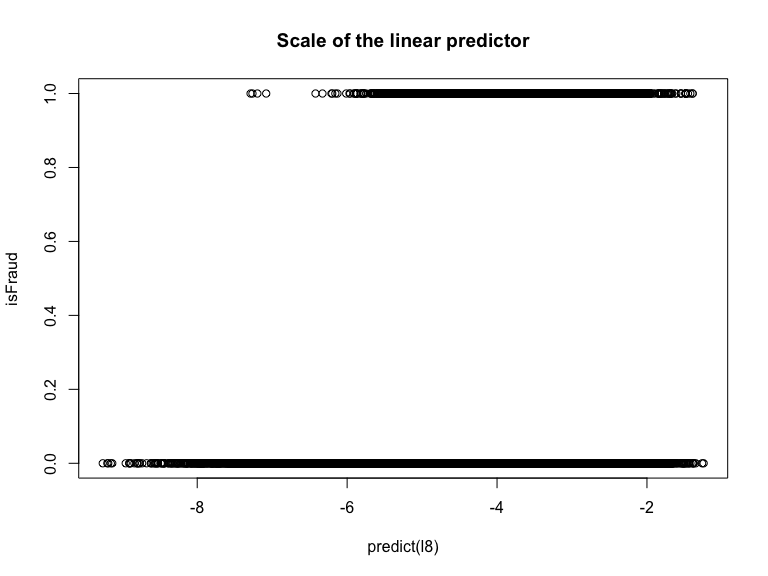source  p=exp(predict(l8))/(1+exp(predict(l8))) plot(p,a$isFraud,main="Scale of p")

plot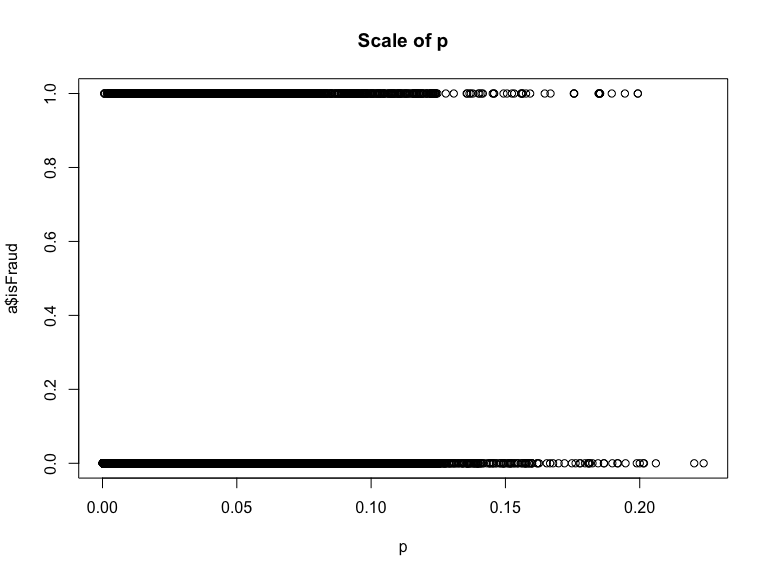Indeed, it is not very easy to understand the possibility of discriminating frauds, from this messy data.

## 1.5 The ROC curve

The ROC curve is built like a parametric curve: for each possible value of the threshold, the True Positive Rate, that is, the Sensitivity of the test, is plotted against the “False Positive Rate” that is, 1-Specifity of the test.

The bisector is the line reflects a random selection to classify units.

source

auc=my.auc(predict(l8),a$isFraud) plot(auc) plot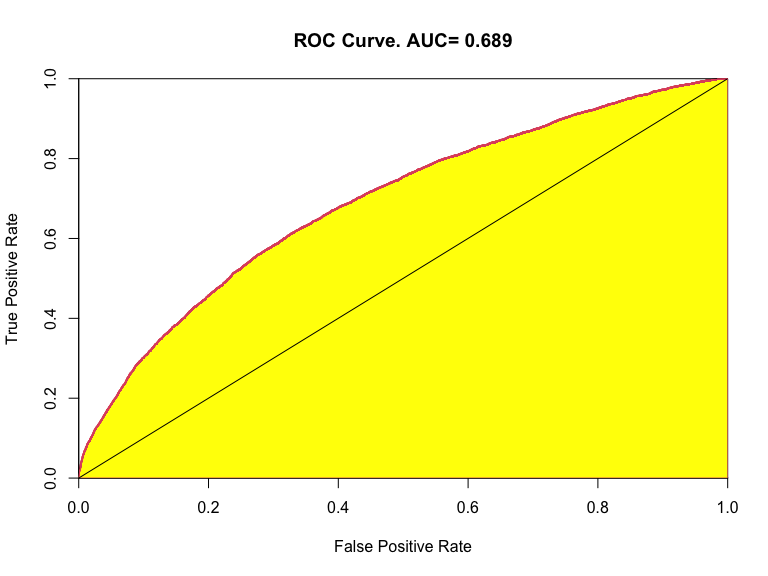In the plot, the ROC curve is the red one (built on the fraud data), the yellow area is the called “Area Under the Curve”. An AUC near to one denotes a very good test, while an area near 0.5 denotes an almost random test. Formally AUC is the probability that two units randomly chosen from the two sets, will be correctly ordered by the predictor test. In our example 0.689 is the probability that if we draw two observations, one fraud and one not fraud, the first has a value of the predictor greater than the second one. ## 1.6 A more complex model source  l9=glm(isFraud~card4*card6+log(TransactionAmt)+I((log(TransactionAmt))^2) +as.factor(hour) +C1*C2+D1*D10*D11*D15,maxit=100,data=a,family=binomial) summary(l9) output  Call: glm(formula = isFraud ~ card4 * card6 + log(TransactionAmt) + I((log(TransactionAmt))^2) + as.factor(hour) + C1 * C2 + D1 * D10 * D11 * D15, family = binomial, data = a, maxit = 100) Deviance Residuals: Min 1Q Median 3Q Max -0.7733 -0.2198 -0.1757 -0.1367 3.8264 Coefficients: Estimate Std. Error z value Pr(>|z|) (Intercept) -6.262e+00 2.832e-01 -22.111 < 2e-16 *** card4mastercard -8.286e-01 8.167e-02 -10.145 < 2e-16 *** card4visa -6.117e-01 7.687e-02 -7.958 1.75e-15 *** card6debit -1.359e+00 5.107e-01 -2.661 0.00780 ** log(TransactionAmt) 1.191e+00 1.060e-01 11.238 < 2e-16 *** I((log(TransactionAmt))^2) -8.618e-02 1.016e-02 -8.486 < 2e-16 *** as.factor(hour)1 -2.873e-02 8.282e-02 -0.347 0.72872 as.factor(hour)2 2.456e-02 8.879e-02 0.277 0.78209 as.factor(hour)3 9.523e-02 9.562e-02 0.996 0.31930 as.factor(hour)4 2.129e-01 1.114e-01 1.912 0.05587 . as.factor(hour)5 7.809e-01 1.156e-01 6.756 1.41e-11 *** as.factor(hour)6 7.895e-01 1.387e-01 5.691 1.26e-08 *** as.factor(hour)7 1.053e+00 1.446e-01 7.285 3.23e-13 *** as.factor(hour)8 8.913e-01 1.738e-01 5.129 2.92e-07 *** as.factor(hour)9 1.072e+00 1.508e-01 7.109 1.17e-12 *** as.factor(hour)10 6.777e-01 1.486e-01 4.561 5.08e-06 *** as.factor(hour)11 4.670e-01 1.197e-01 3.902 9.53e-05 *** as.factor(hour)12 4.160e-01 9.526e-02 4.368 1.26e-05 *** as.factor(hour)13 7.182e-02 8.999e-02 0.798 0.42480 as.factor(hour)14 4.375e-02 8.288e-02 0.528 0.59756 as.factor(hour)15 8.874e-03 7.984e-02 0.111 0.91150 as.factor(hour)16 1.125e-01 7.487e-02 1.502 0.13301 as.factor(hour)17 1.343e-01 7.332e-02 1.831 0.06707 . as.factor(hour)18 2.017e-01 7.198e-02 2.802 0.00508 ** as.factor(hour)19 2.163e-01 7.166e-02 3.019 0.00254 ** as.factor(hour)20 1.402e-01 7.314e-02 1.916 0.05531 . as.factor(hour)21 9.835e-02 7.358e-02 1.337 0.18134 as.factor(hour)22 3.080e-02 7.446e-02 0.414 0.67912 as.factor(hour)23 2.300e-01 7.258e-02 3.170 0.00153 ** C1 -6.397e-02 5.588e-03 -11.447 < 2e-16 *** C2 7.073e-02 5.531e-03 12.788 < 2e-16 *** D1 -3.201e-03 5.291e-04 -6.050 1.44e-09 *** D10 1.137e-03 2.239e-04 5.079 3.80e-07 *** D11 7.472e-04 2.501e-04 2.987 0.00281 ** D15 -4.180e-04 2.246e-04 -1.861 0.06279 . card4mastercard:card6debit 5.728e-01 5.133e-01 1.116 0.26446 card4visa:card6debit 7.511e-01 5.119e-01 1.467 0.14232 C1:C2 -3.095e-05 1.795e-05 -1.724 0.08473 . D1:D10 -1.613e-06 2.108e-06 -0.765 0.44433 D1:D11 -3.266e-06 2.388e-06 -1.368 0.17137 D10:D11 -7.853e-06 1.524e-06 -5.152 2.58e-07 *** D1:D15 6.879e-06 1.224e-06 5.618 1.93e-08 *** D10:D15 -5.653e-07 7.021e-07 -0.805 0.42067 D11:D15 -4.761e-06 8.910e-07 -5.343 9.13e-08 *** D1:D10:D11 1.118e-08 8.004e-09 1.397 0.16231 D1:D10:D15 -2.919e-09 4.194e-09 -0.696 0.48641 D1:D11:D15 6.762e-09 4.618e-09 1.464 0.14317 D10:D11:D15 1.423e-08 3.160e-09 4.502 6.72e-06 *** D1:D10:D11:D15 -2.341e-11 1.457e-11 -1.607 0.10810 --- Signif. codes: 0 '***' 0.001 '**' 0.01 '*' 0.05 '.' 0.1 ' ' 1 (Dispersion parameter for binomial family taken to be 1) Null deviance: 59702 on 308895 degrees of freedom Residual deviance: 56601 on 308847 degrees of freedom AIC: 56699 Number of Fisher Scoring iterations: 8 source  AIC(l9) output  56698.68 source auc=my.auc(predict(l9),a$isFraud)
plot(auc)

plot